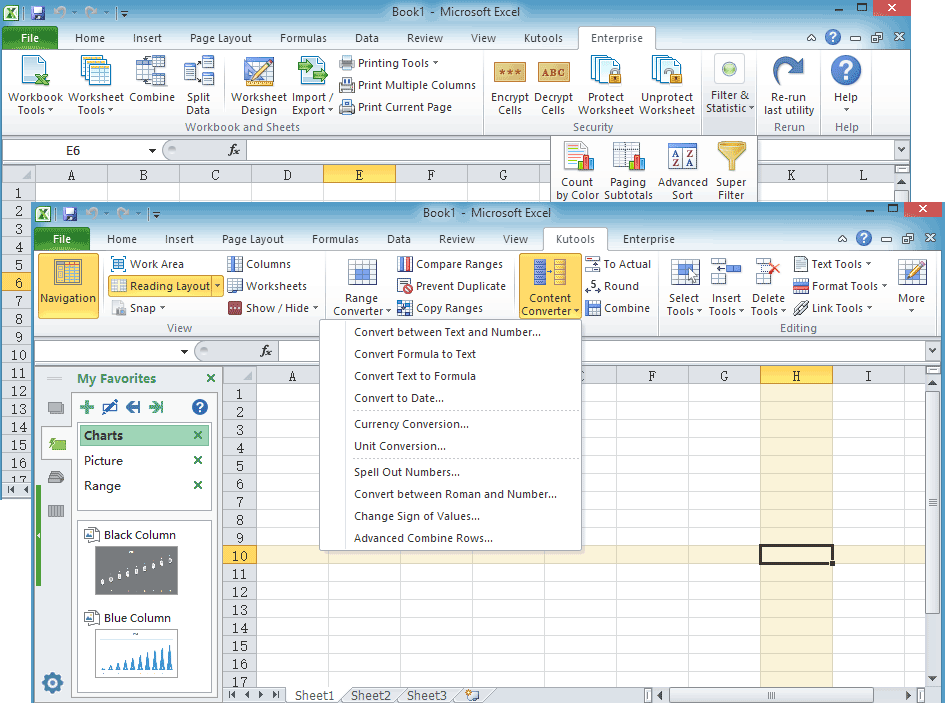Note: The other languages of the website are Google-translated. Back to English

## Find the largest value smaller than a specified number in Excel

### Kutools for Excel

In Excel, you can use the formula MAX() to find the largest value from a range, but if you want to find the largest value which is smaller than a certain number, which formula can you use? In Kutools for Excel, the powerful Formula Helper provides a formula Find the largest value less than can handle this job and let you free from remembering formulas.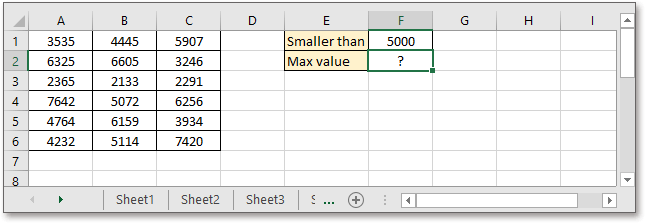#### Click Kutools > Formula Helper > Formula Helper, then in the popping Formulas Helper dialog, choose Lookup from the Formula Type drop-down list, and select Find the largest value less than from the list in Choose a formula section. See screenshot: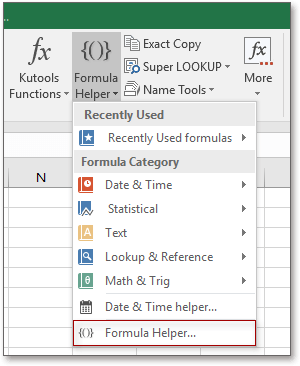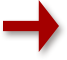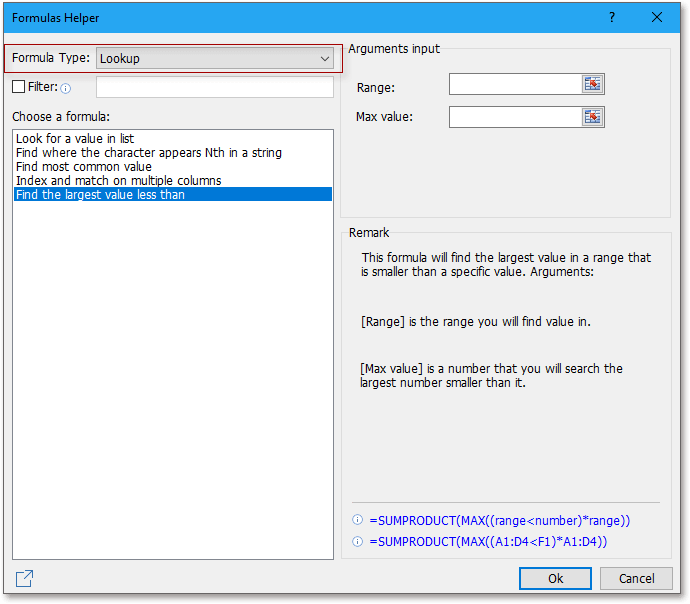To find the largest value but smaller than a number, please do as below:

1. Select cell to place the formula, then click Kutools > Formula Helper > Formula Helper.2. In the Formulas Helper dialog, choose Lookup from the drop-down list of Formula Type, then go down to select Find the largest value less than in the Choose a formula section.3. Then in the Arguments input section, click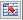to select the range cells you want to find value from, and the maximum value you specified.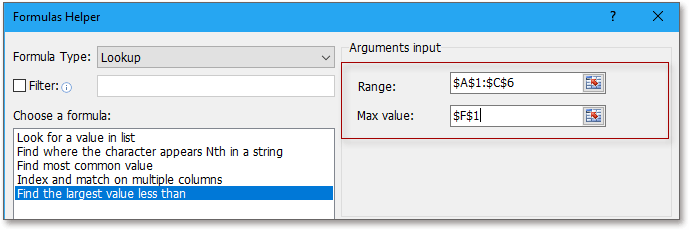4. Click Ok, then the largest value which is less than the specified number has been gotten.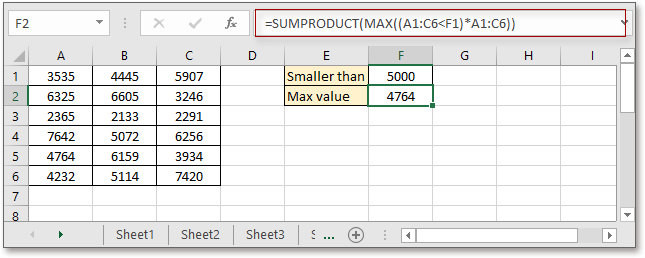Notes:

1. In the Formula Helper dialog, you can check Filter checkbox, then in the textbox, type the keyword to search formula in the Formulas Helper dialog.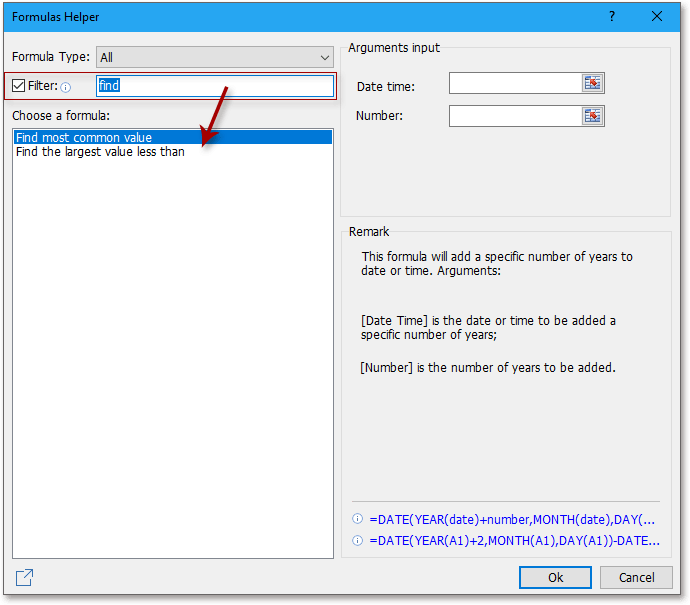2. You can directly type the max value into the textbox in the Argument input section.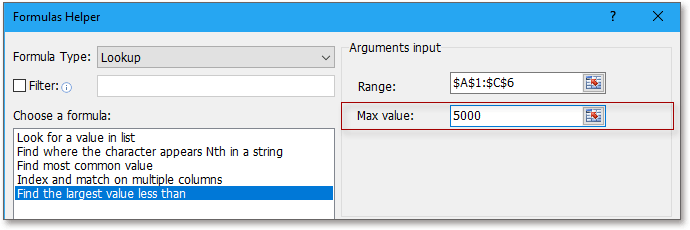3. If this feature does you a favor, you could click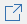to share this feature to your friends.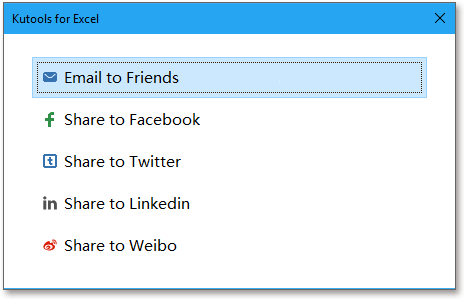Productivity Tools Recommended
The following tools can greatly save your time and money, which one is right for you?
Office Tab: Using handy tabs in your Office, as the way of Chrome, Firefox and New Internet Explorer.
Kutools for Excel: More than 300 Advanced Functions for Excel 2021, 2019, 2016, 2013, 2010, 2007 and Office 365.

### Kutools for Excel

The functionality described above is just one of 300 powerful functions of Kutools for Excel.

Designed for Excel(Office) 2021, 2019, 2016, 2013, 2010, 2007 and Office 365. Free download and use for 60 days.